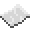# math.mod

Return the remainder of dividing x by y. This has the same behaviour as the `%` operator.ExampleTest if user input is even
Read a line from the user, then print if it is evenly divisible by 2.
Code
```<nowiki>
</nowiki>
```
Output Depends on what the user wrote. For instance, if they were to enter `5`, the value printed would be `false`.

math.mod
Function
Syntax
math.mod(
• x : number
• y : number
)

Returns number
API math
Source Lua (source)